# Intermediate Maths Solutions for 1A Exercise 6(f) Trigonometric Ratios Upto Transformations

Inter Maths 1A Solutions for exercise 6(f) Trigonometeic Ratios Upto Transformations are given.

These solutions are very easy to understand. Please study the text book lesson very well.

Observe the problems and solutions given in the text book. Try them well.

Observe the given solutions and try them in your own method.

You can see the solutions for Maths 1A text book

You can also see

Inter Maths 1A textbook solutions

Inter Maths 1B textbook solutions

Inter Maths IIA textbook solutions

Inter Maths IIB textbook solutions

Trigonometric Ratios upto Transformations

Exercise 6(a)

Exercise 6(b)

Exercise 6(c)

Exercise 6(d)

Exercise 6(e)

Inter Maths Trigonometry text book solutions

Nios Solutions for Maths 311 Book 1 Sets 1.1

M

# Inter Maths solutions for Trigonometric Ratios upto Transformations Exercise 6(f)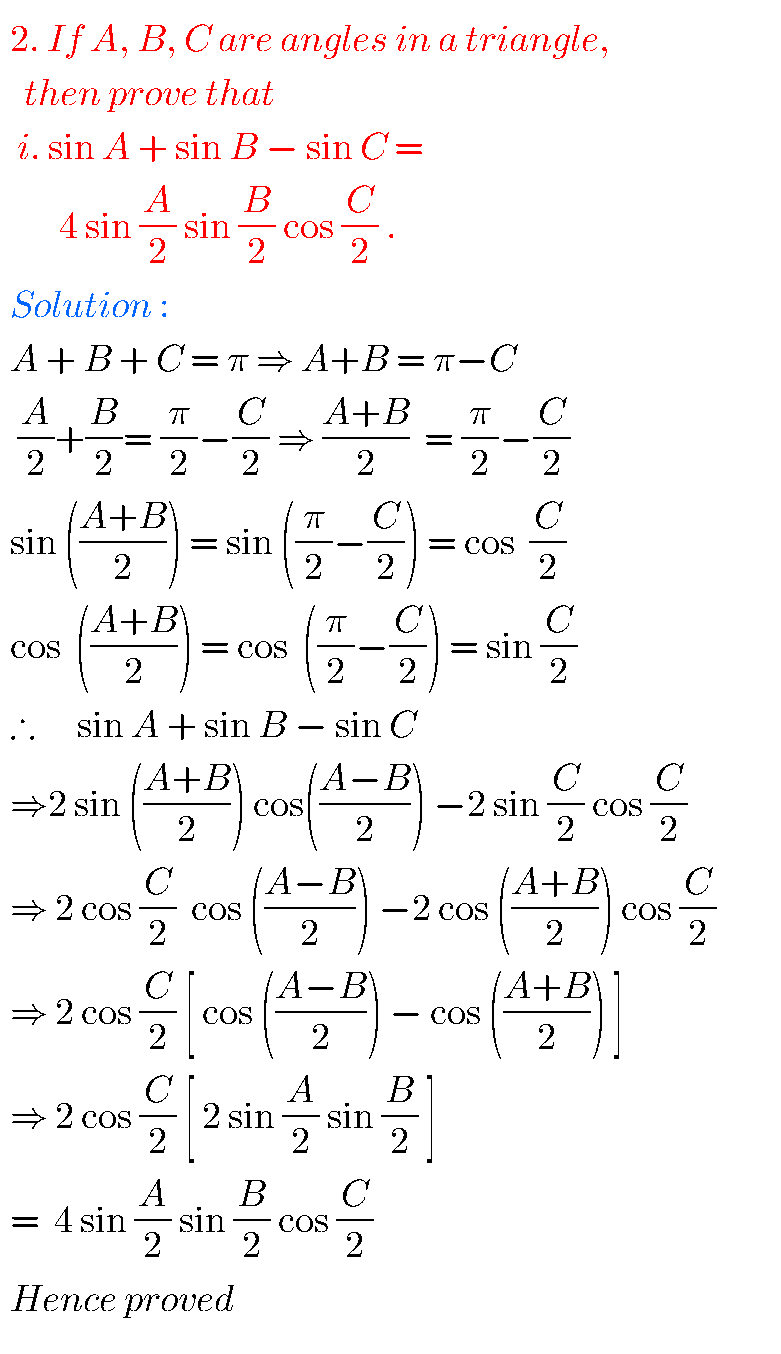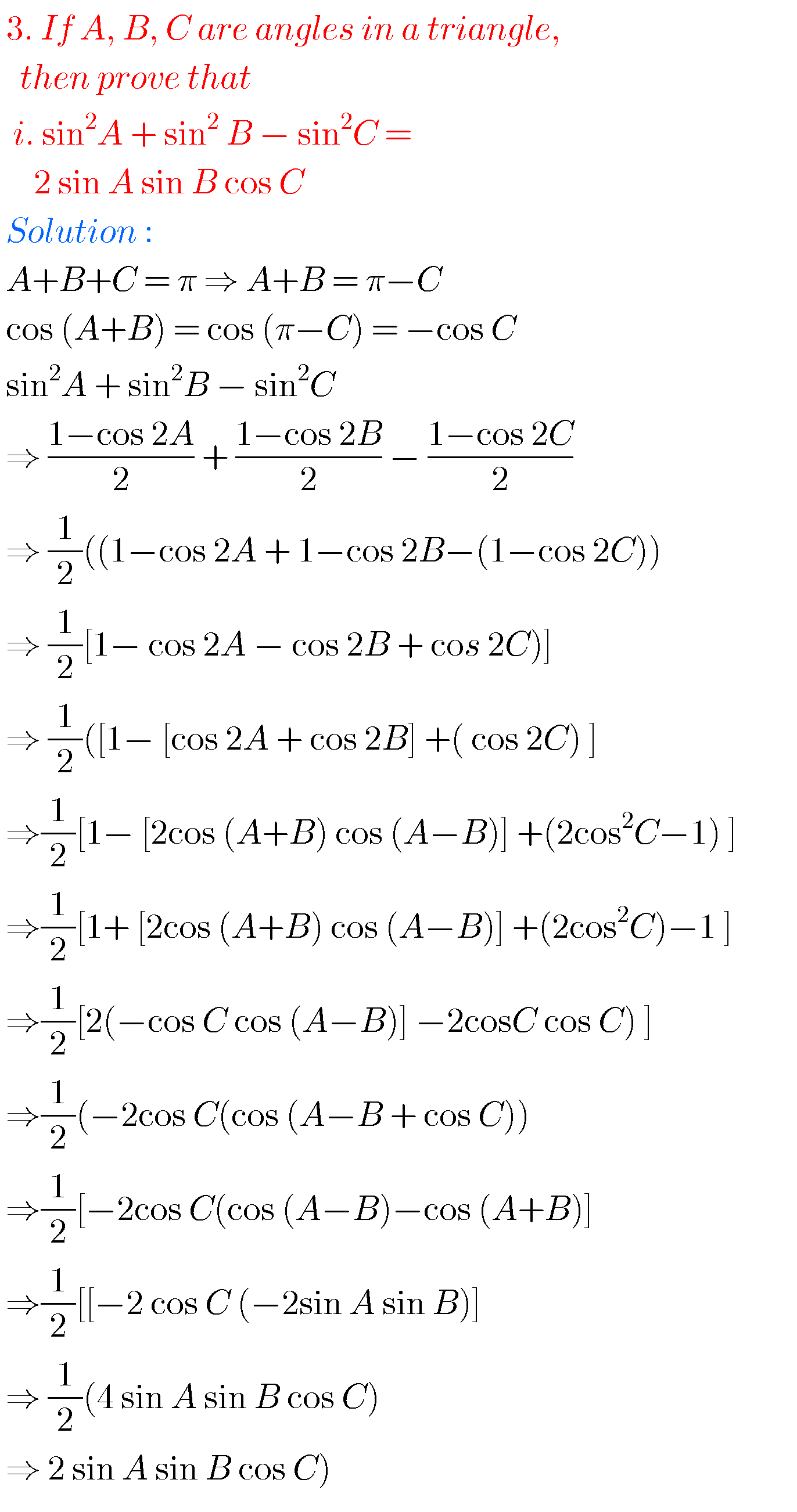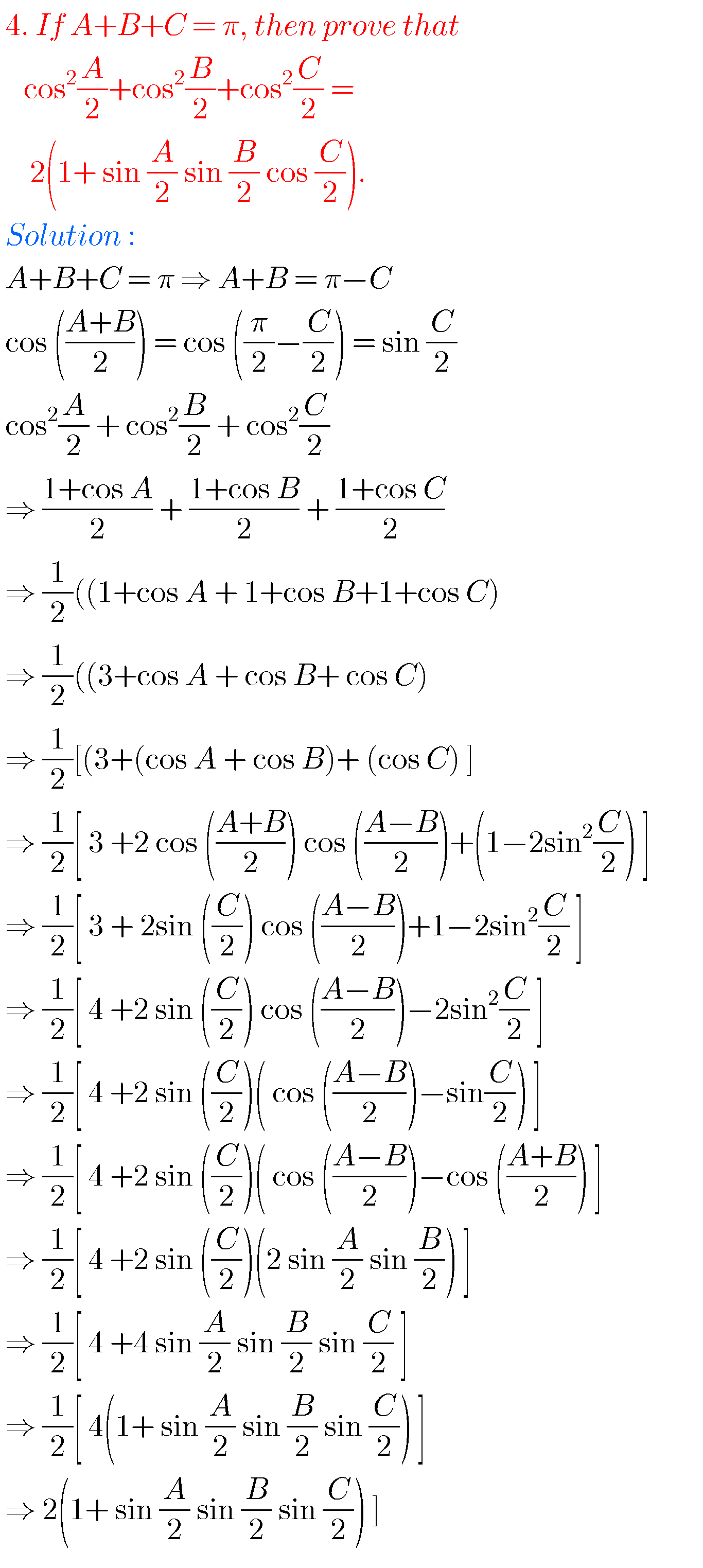## Trigonometric Ratios upto Transformations solutions Inter exercise 6(f)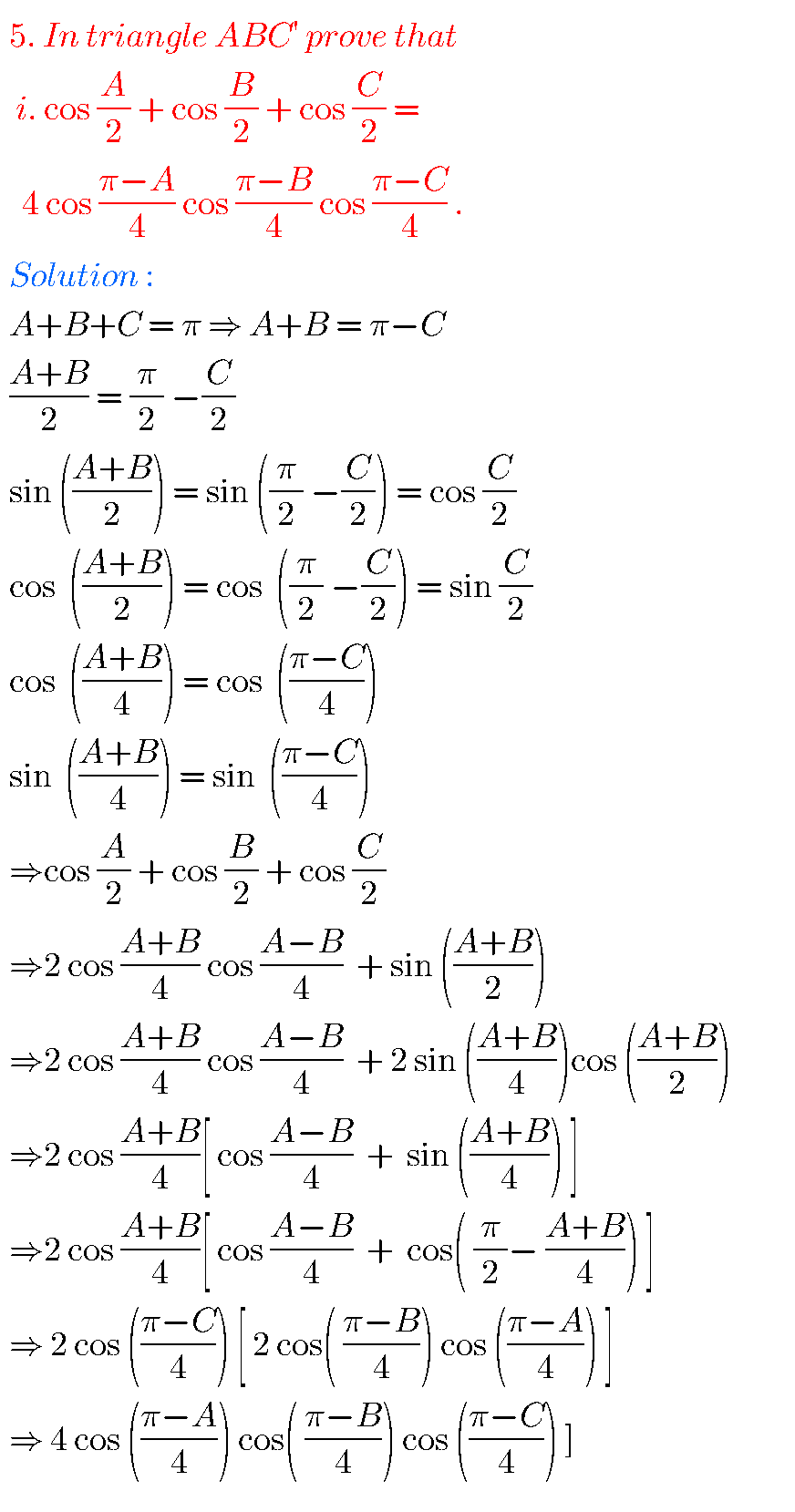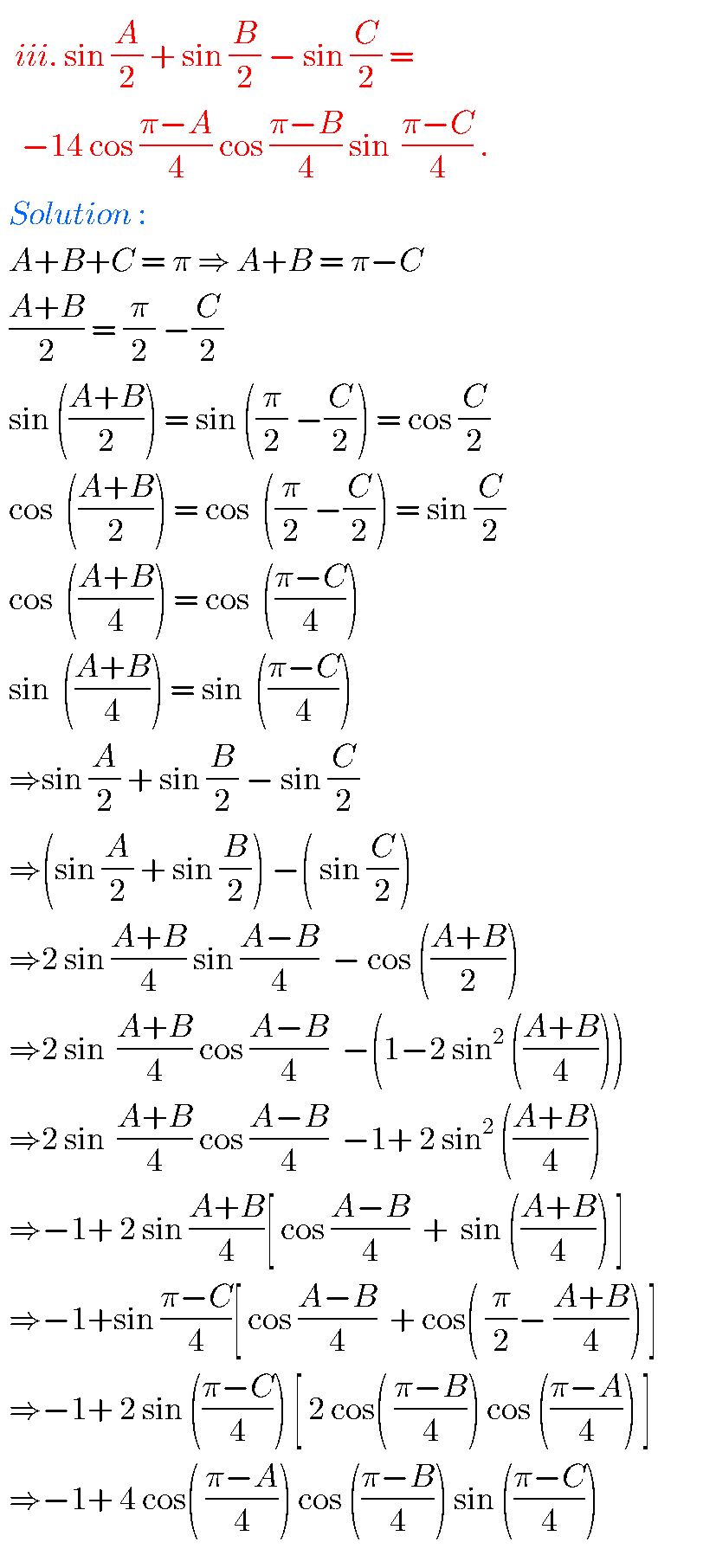## Maths 1A Inter Trigonometric Ratios upto Transformations exercise 6(f) solutions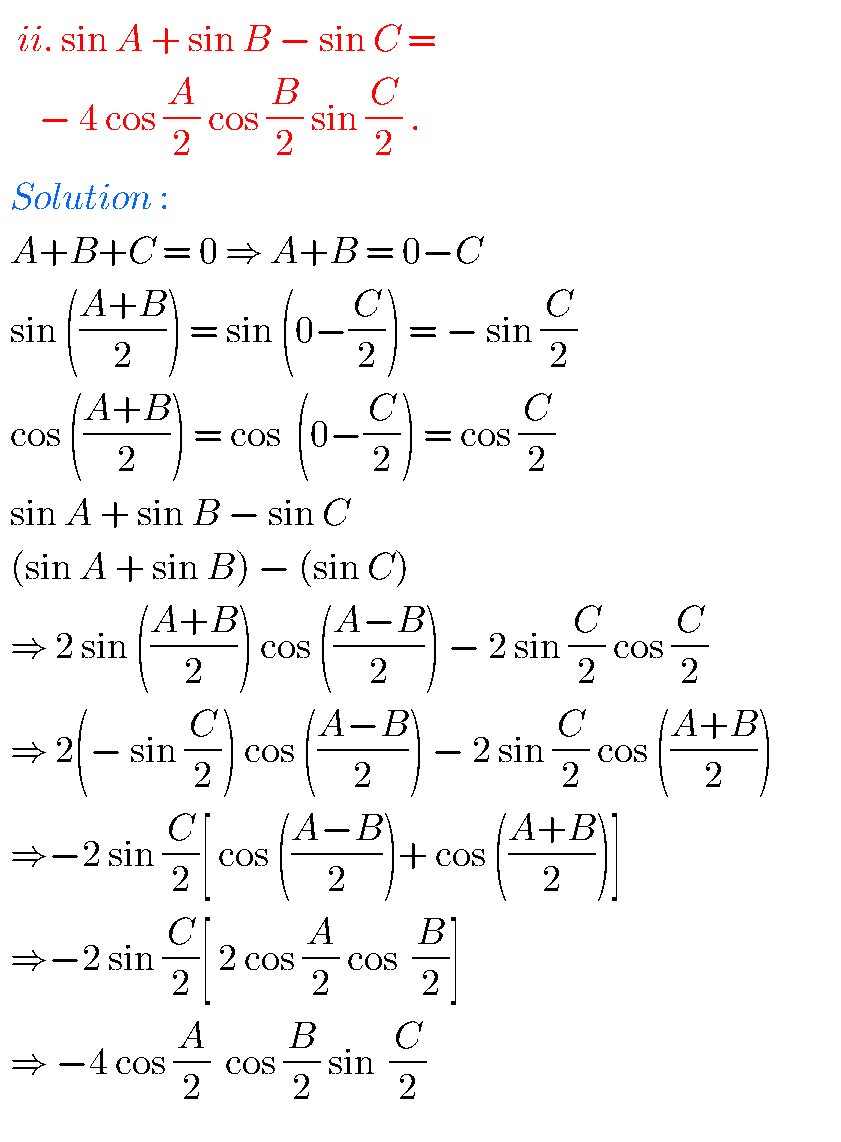Note : Observe the solutions and try them in your own method.

You can see the solutions for Inter maths 1A for examination purpose

3. Matrices

Trigonometric ratios upto transformations 2

You can also see the solutions

SSC Maths Solutions

Ncert solutions for Principle of Mathematical Induction

Ncert solutions for Linear Equations in two variables class 8

Ncert solutions for Simple Equations class 7

Ncert maths class 6 Fractions exercise 7.2 solutions

### 1 thought on “Intermediate Maths Solutions for 1A Exercise 6(f) Trigonometric Ratios Upto Transformations”

1.Doing good. As q guide it is so useful❤